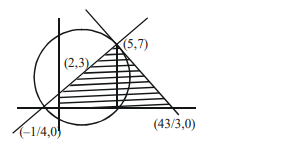# If the area of the triangle formed by the positive

Question:

If the area of the triangle formed by the positive $x$-axis, the normal and the tangent to the circle $(x-2)^{2}+(y-3)^{2}=25$ at the point $(5,7)$ is $A$, then $24 \mathrm{~A}$ is equal to

Solution:Equation of normal

$4 x-3 y+1=0$

and equation of tangents

$3 x+4 y-43=0$

Area of triangle $=\frac{1}{2}\left(\frac{43}{3}+\frac{1}{4}\right) \times(7)$

$=\frac{1}{2}\left(\frac{172+3}{12}\right) \times 7$

$A=\frac{1225}{24}$

$24 \mathrm{~A}=1225$

* as positive $x$-axis is given in the question so question should be bonus.